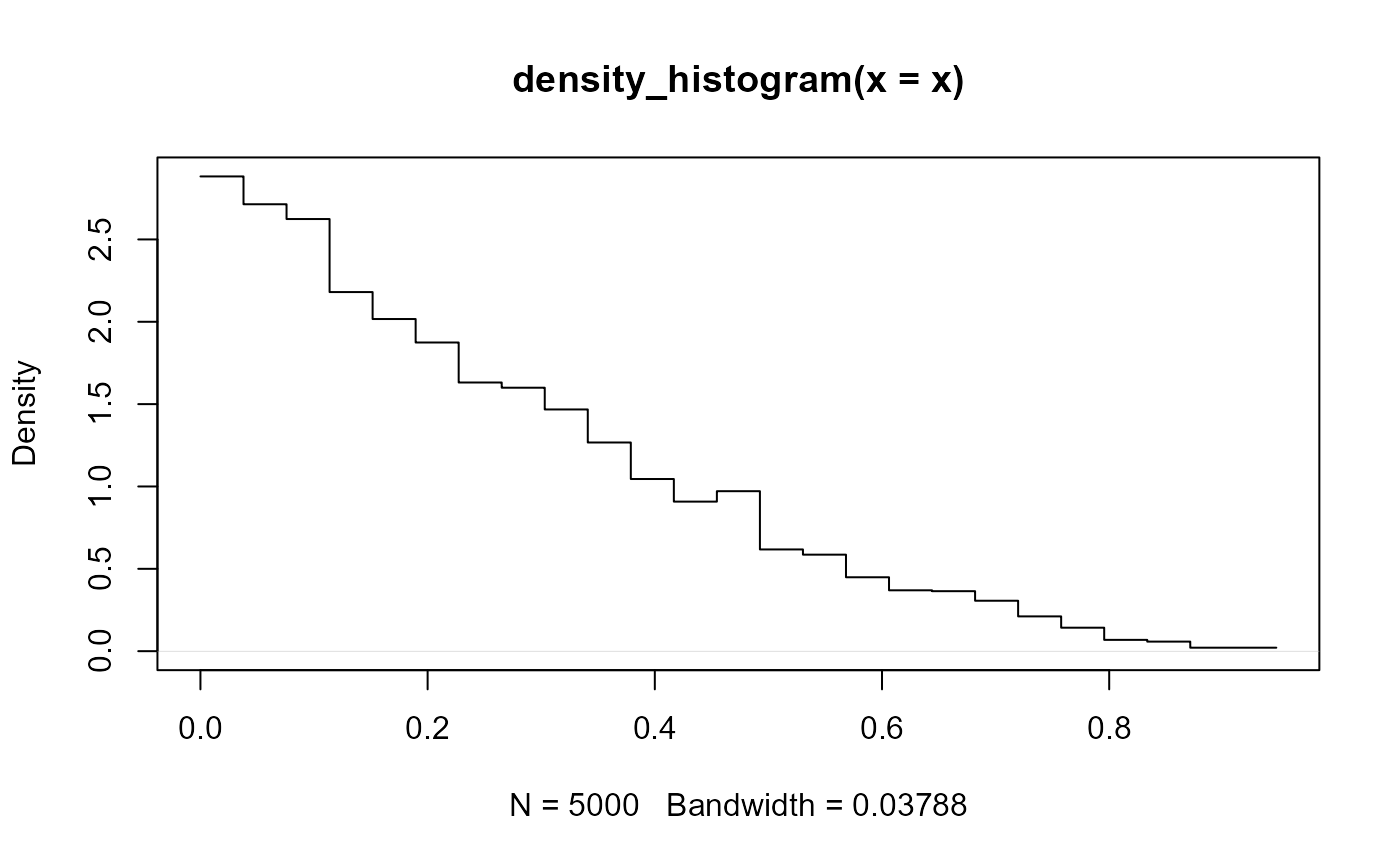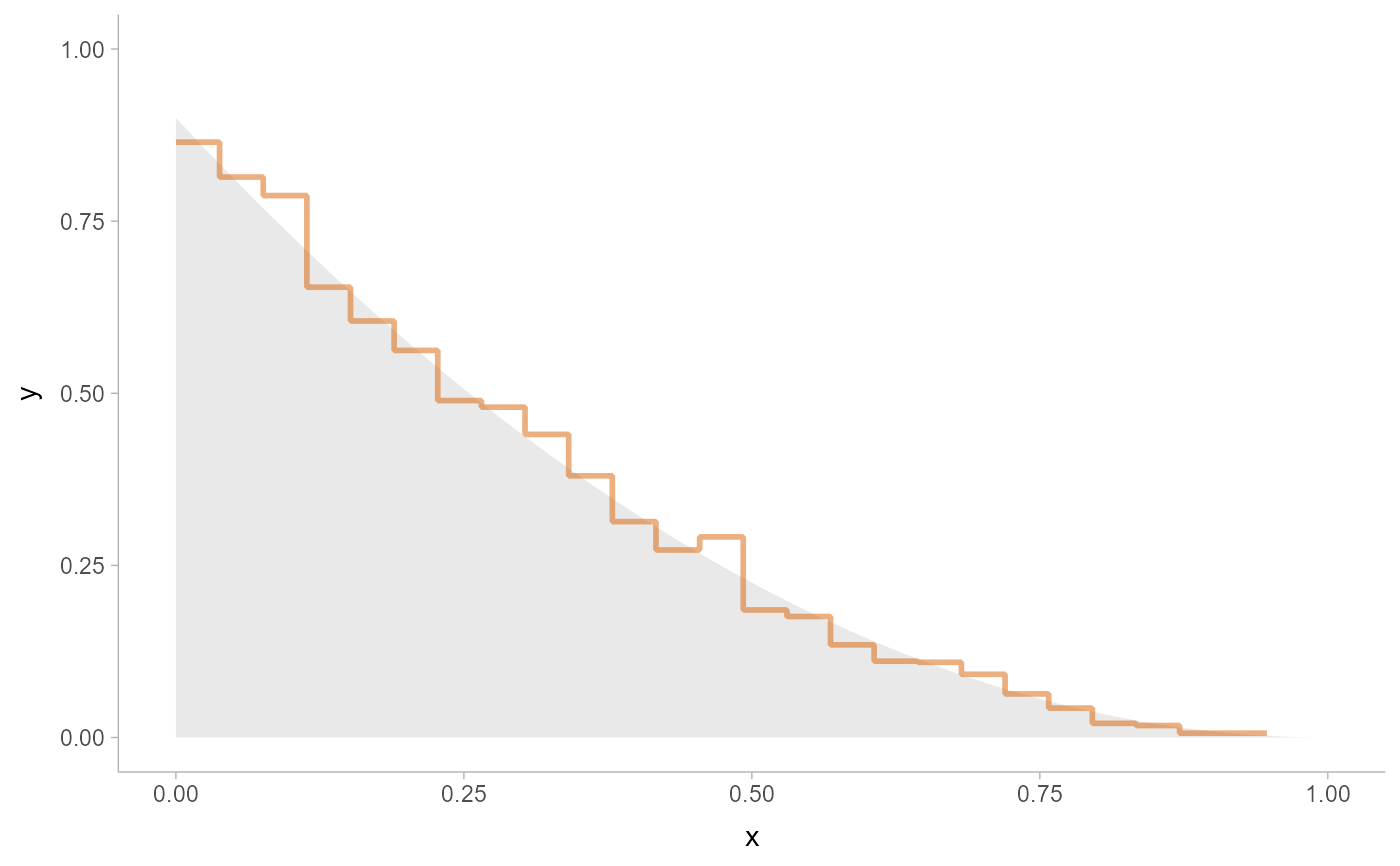Histogram density estimator. Supports automatic partial function application.

density_histogram(
x,
weights = NULL,
breaks = "Sturges",
align = "none",
outline_bars = FALSE,
na.rm = FALSE,
...,
range_only = FALSE
)

## Arguments

x

numeric vector containing a sample to compute a density estimate for.

weights

optional numeric vector of weights to apply to x.

breaks

Determines the breakpoints defining bins. Similar to (but not exactly the same as) the breaks argument to graphics::hist(). One of:

• A scalar (length-1) numeric giving the number of bins

• A vector numeric giving the breakpoints between histogram bins

• A function taking x and weights and returning either the number of bins or a vector of breakpoints

• A string giving the suffix of a function that starts with "breaks_". ggdist provides weighted implementations of the "Sturges", "Scott", and "FD" break-finding algorithms from graphics::hist(), as well as breaks_fixed() for manually setting the bin width. See breaks.

For example, breaks = "Sturges" will use the breaks_Sturges() algorithm, breaks = 9 will create 9 bins, and breaks = breaks_fixed(width = 1) will set the bin width to 1.

align

Determines how to align the breakpoints defining bins. One of:

• A scalar (length-1) numeric giving an offset that is subtracted from the breaks. The offset must be between 0 and the bin width.

• A function taking a sorted vector of breaks (bin edges) and returning an offset to subtract from the breaks.

• A string giving the suffix of a function that starts with "align_" used to determine the alignment, such as align_none(), align_boundary(), or align_center().

For example, align = "none" will provide no alignment, align = align_center(at = 0) will center a bin on 0, and align = align_boundary(at = 0) will align a bin edge on 0.

outline_bars

Should outlines in between the bars (i.e. density values of 0) be included?

na.rm

Should missing (NA) values in x be removed?

...

range_only

If TRUE, the range of the output of this density estimator is computed and is returned in the $x element of the result, and c(NA, NA) is returned in $y. This gives a faster way to determine the range of the output than density_XXX(n = 2).

## Value

An object of class "density", mimicking the output format of stats::density(), with the following components:

• x: The grid of points at which the density was estimated.

• y: The estimated density values.

• bw: The bandwidth.

• n: The sample size of the x input argument.

• call: The call used to produce the result, as a quoted expression.

• data.name: The deparsed name of the x input argument.

• has.na: Always FALSE (for compatibility).

• cdf: Values of the (possibly weighted) empirical cumulative distribution function at x. See weighted_ecdf().

This allows existing methods for density objects, like print() and plot(), to work if desired. This output format (and in particular, the x and y components) is also the format expected by the density argument of the stat_slabinterval()

and the smooth_ family of functions.

Other density estimators: density_bounded(), density_unbounded()

## Examples

library(distributional)
library(dplyr)
library(ggplot2)

# For compatibility with existing code, the return type of density_unbounded()
# is the same as stats::density(), ...
set.seed(123)
x = rbeta(5000, 1, 3)
d = density_histogram(x)
d
#>
#> Call:
#> 	density_histogram(x = x)
#>
#> Data: x (5000 obs.);	Bandwidth 'bw' = 0.07285
#>
#>        x                   y
#>  Min.   :0.0000338   Min.   :0.02196
#>  1st Qu.:0.2277000   1st Qu.:0.31845
#>  Median :0.4735795   Median :0.86475
#>  Mean   :0.4735795   Mean   :1.05586
#>  3rd Qu.:0.7194591   3rd Qu.:1.62244
#>  Max.   :0.9471253   Max.   :2.82486

# ... thus, while designed for use with the density argument of
# stat_slabinterval(), output from density_histogram() can also be used with
# base::plot():
plot(d)# here we'll use the same data as above with stat_slab():
data.frame(x) %>%
ggplot() +
stat_slab(
aes(xdist = dist), data = data.frame(dist = dist_beta(1, 3)),
alpha = 0.25
) +
stat_slab(aes(x), density = "histogram", fill = NA, color = "#d95f02", alpha = 0.5) +
scale_thickness_shared() +
theme_ggdist()